# 技术实操丨使用ModelArts和HiLens Studio完成云端验证及部署

【摘要】 华为HiLens，是面向AI开发者、企业、硬件厂商的端云协同AI平台，由具备AI能力的摄像头和云上开发平台组成，包括一站式技能开发、设备安装与管理、数据管理、技能市场等，帮助用户开发AI技能并将其赋予端侧计算设备。

### 前言

HiLens Studio公测也出来一阵子了，亮点很多，我前些天也申请了公测，通过后赶快尝试了一下，不得不说真的很不错啊，特别是支持云端编辑代码，调试，甚至可以直接运行程序，即使自己的HiLens不在身边，也可以得到程序运行结果，不仅仅是云端IDE这么简单，更是有云端硬件资源支撑，极大降低了开发者负担，开发者只需要一台可以联网的电脑就行了，可以快速验证，验证通过后，直接安装到自己的HiLens上就能应用了，真是太棒了。

### 正文

1. 创建数据集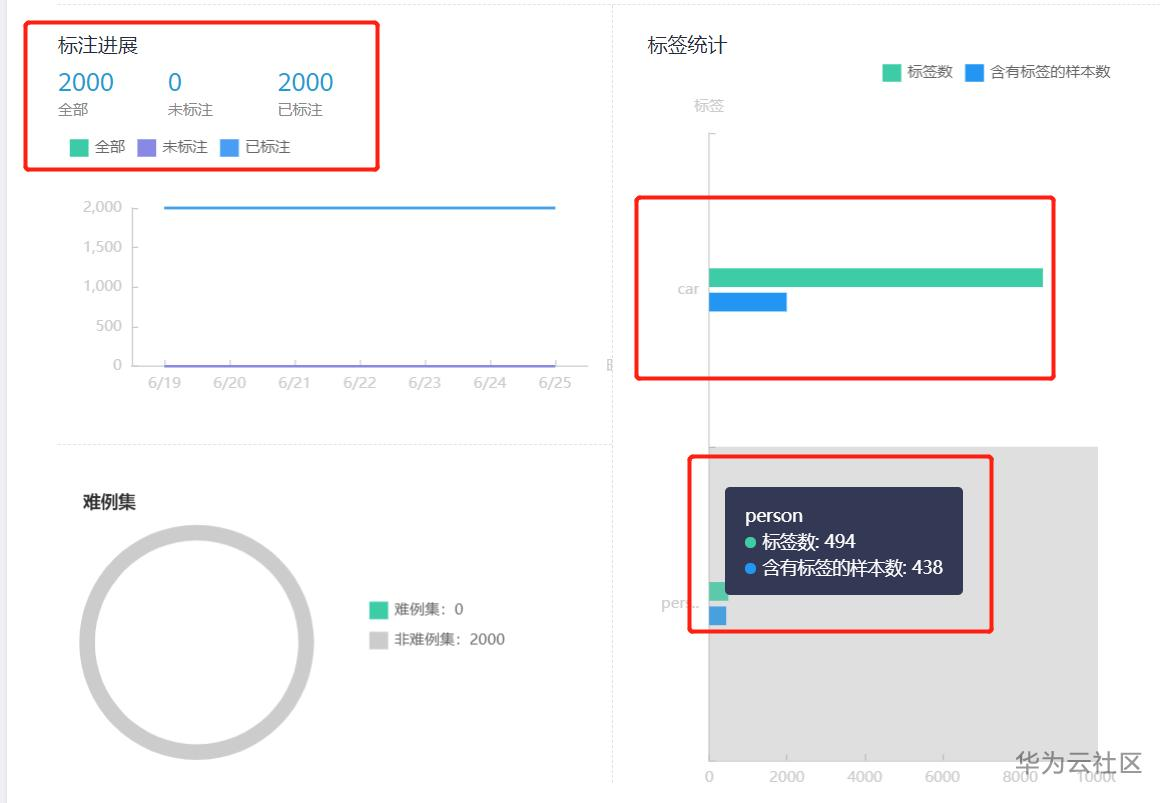2. 模型训练

3. 模型转换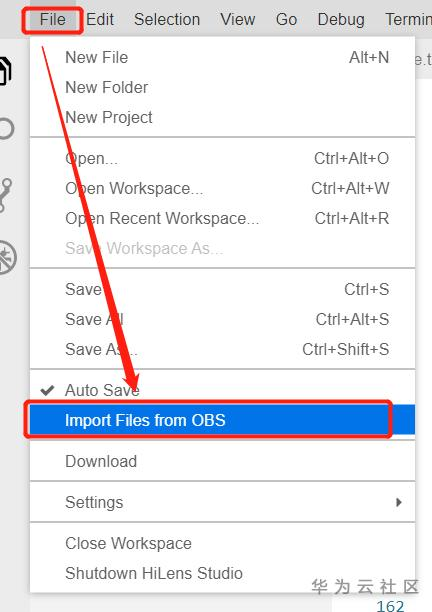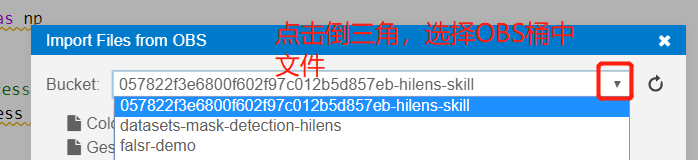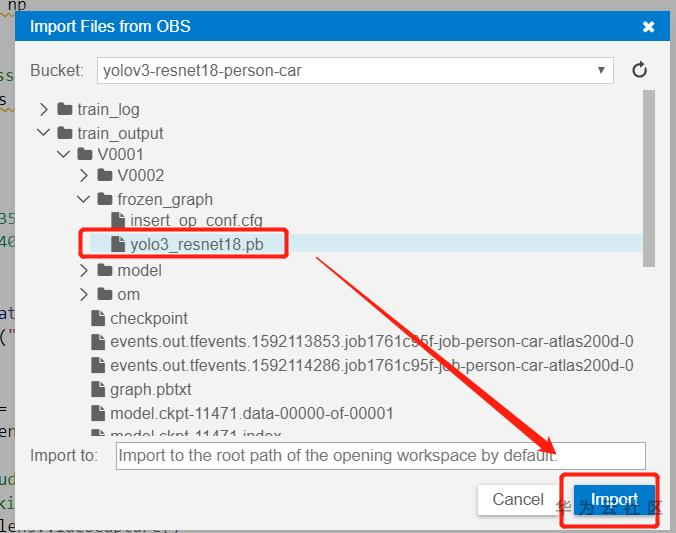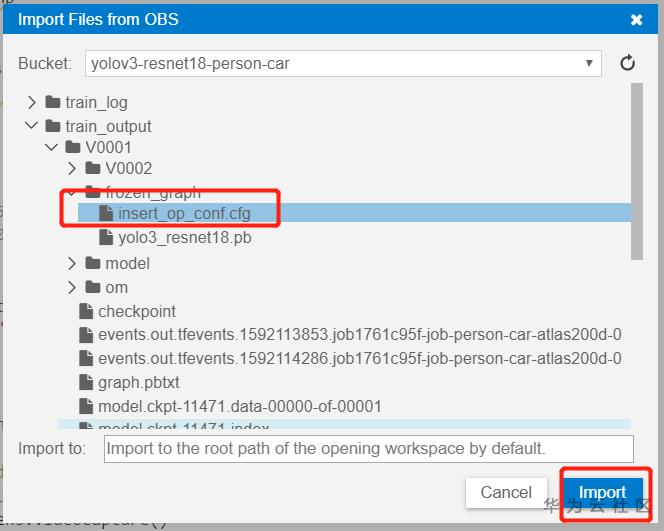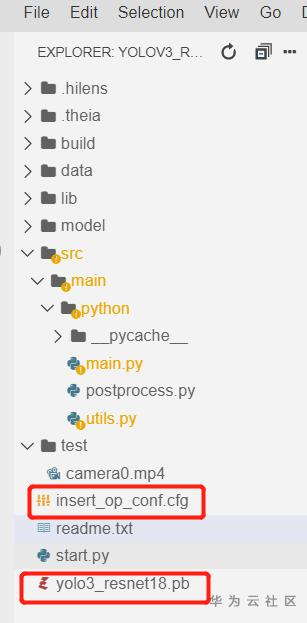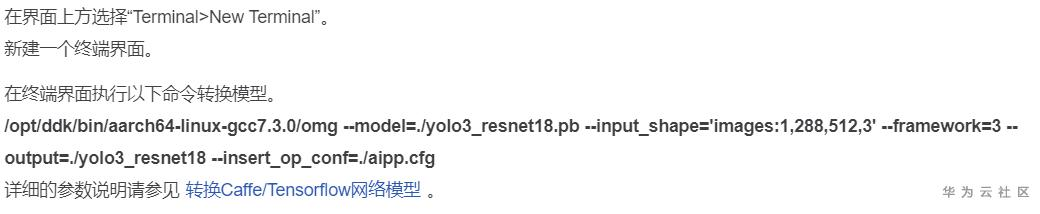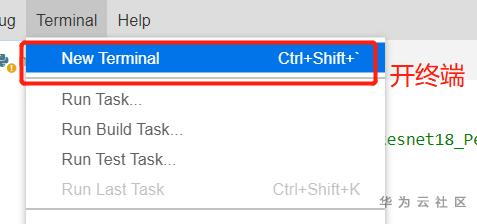```/opt/ddk/bin/aarch64-linux-gcc7.3.0/omg --model=./yolo3_resnet18.pb --input_shape='images:1,352,640,3' --framework=3 --output=./yolo3_resnet18
--insert_op_conf=./insert_op_conf.cfg```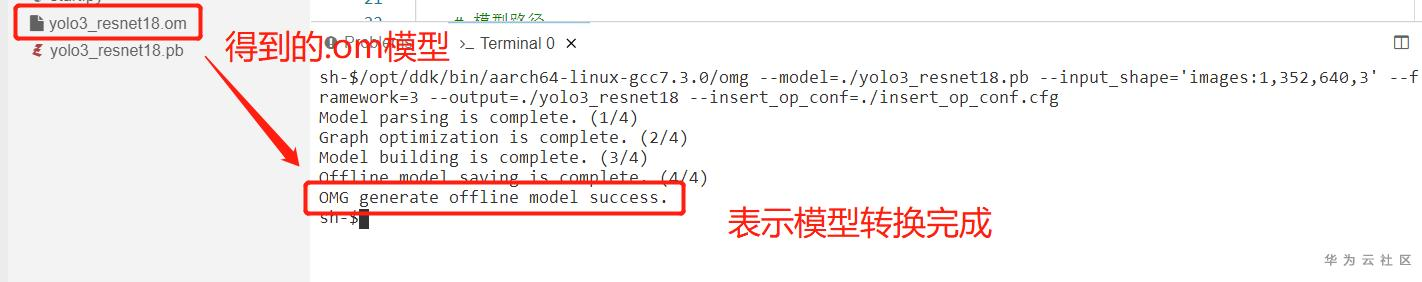https://console.huaweicloud.com/modelarts/?region=cn-north-4#/aiMarket/aiMarketModelDetail/overview?modelId=7087008a-7eec-4977-8b66-3a7703e9fd22&type=algo

https://www.huaweicloud.com/ascend/doc/Atlas200DK/1.31.0.0(beta)/zh/zh-cn_topic_0211633857.html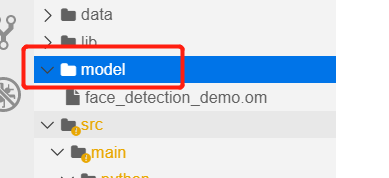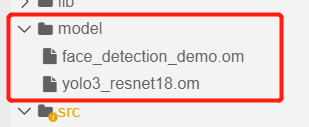### 如果你不想自己训练，只是测试一下，这里提供了转换完成的.om模型，下载后，上传到HiLens Studio的model文件夹下即可使用：

4. 编辑代码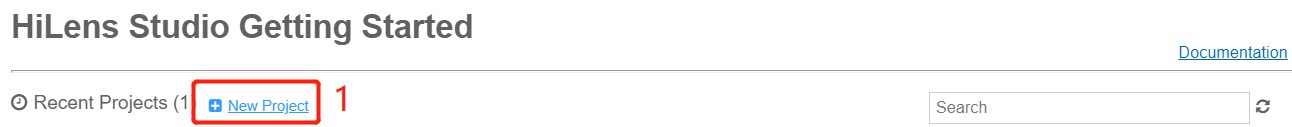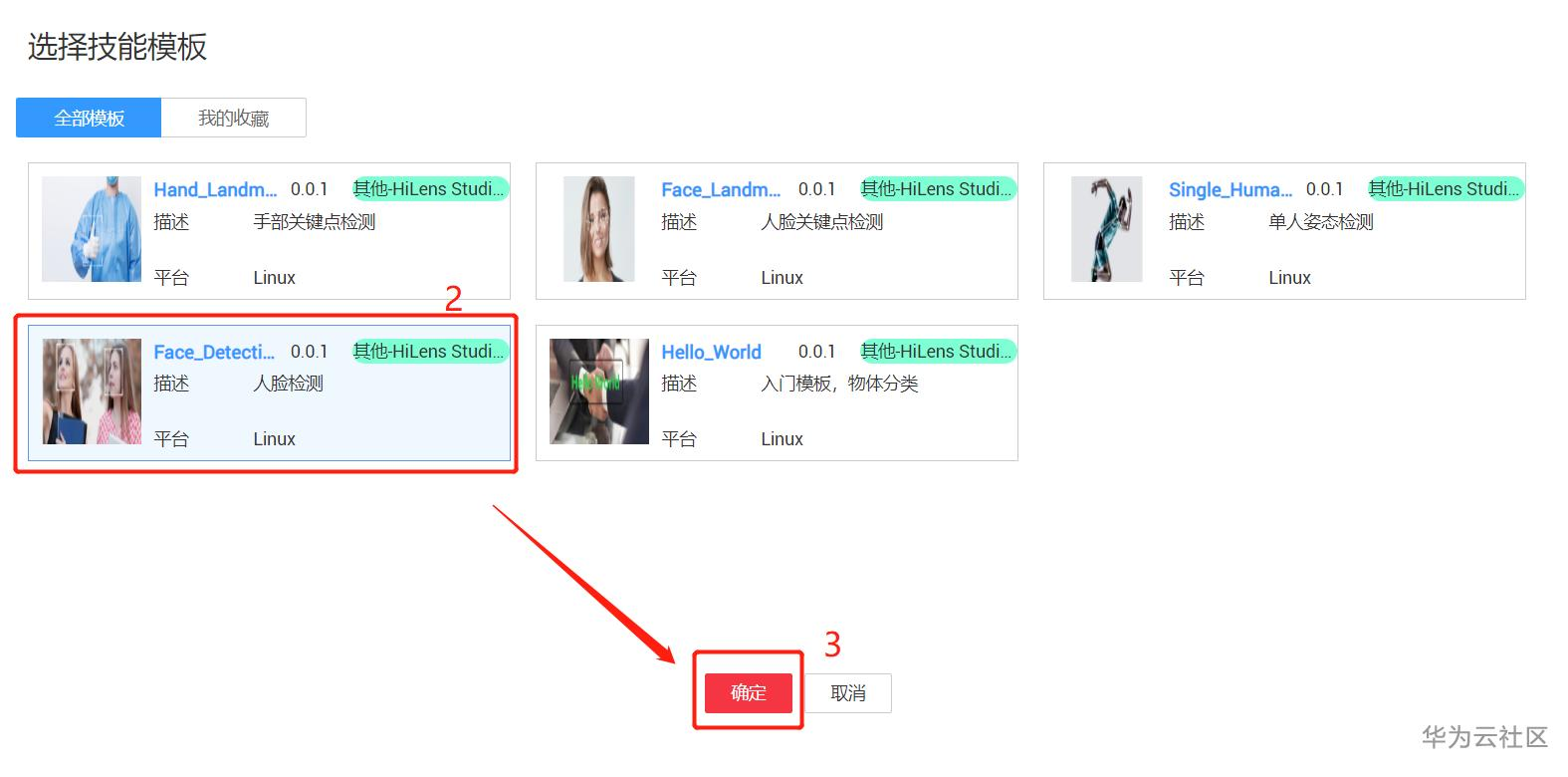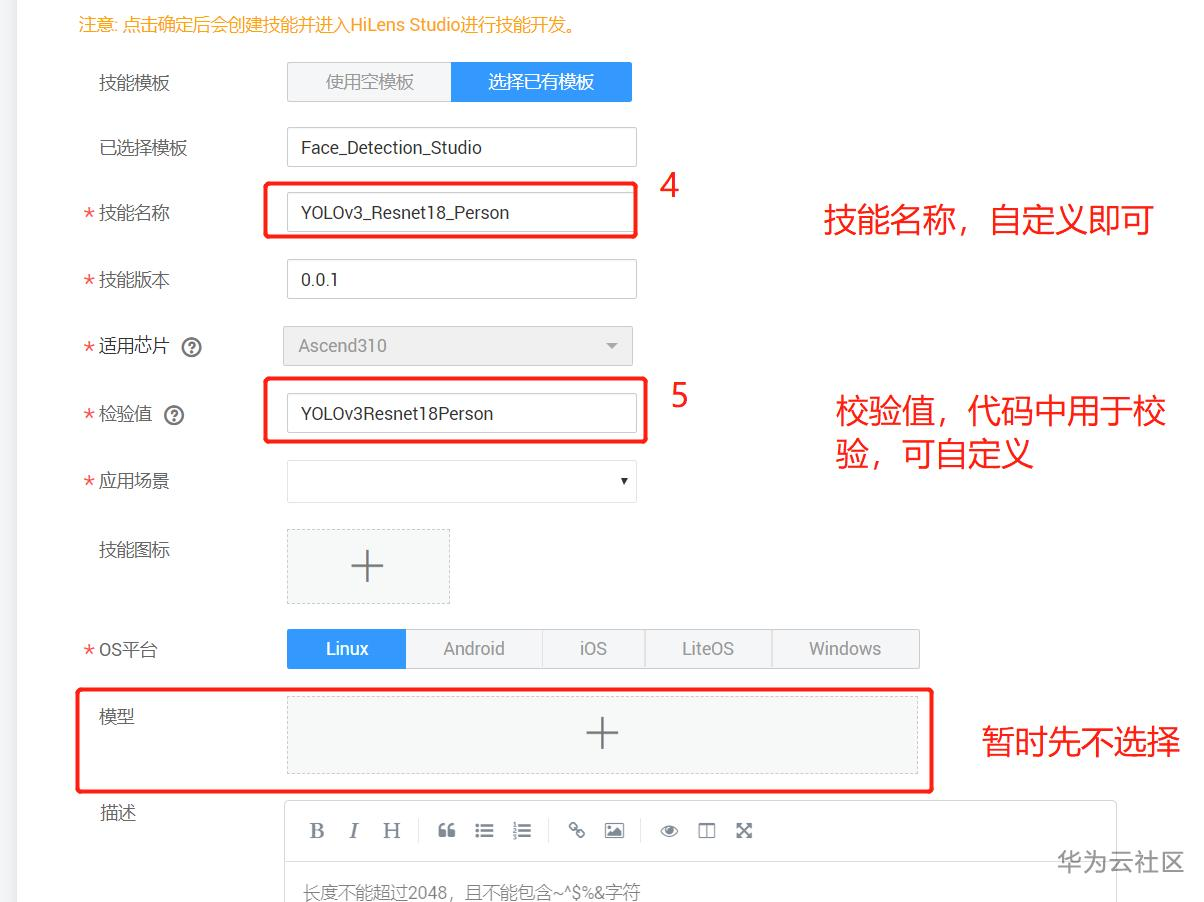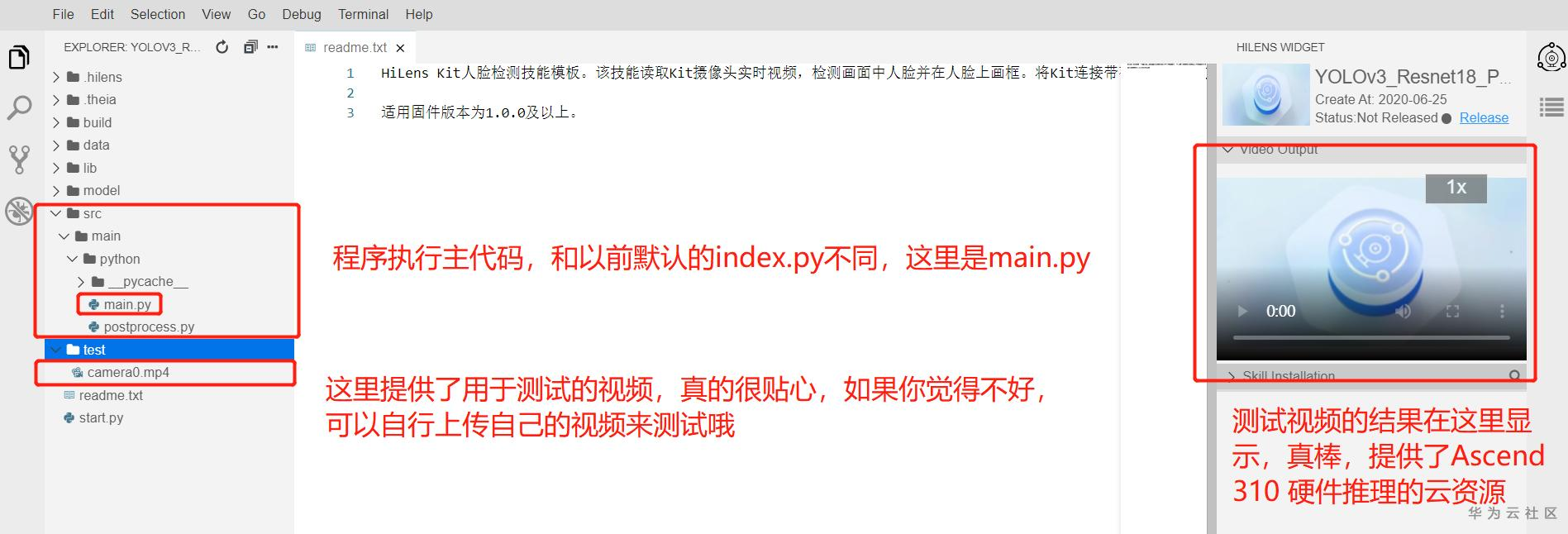main.py主代码

```# -*- coding: utf-8 -*-
# !/usr/bin/python3
# SkillFramework 1.0.0 YOLOv3_Resnet18_Person

import cv2
import numpy as np
import os
import hilens
# 这个postprocess没用哈
from postprocess import im_detect_nms
import utils

# 网络输入尺寸
input_height = 352
input_width = 640

def main(work_path):
hilens.init("YOLOv3Resnet18Person")  # 参数要与创建技能时填写的检验值保持一致！

# 模型路径
model_path = os.path.join(work_path, 'model/yolo3_resnet18.om')
model = hilens.Model(model_path)

# hilens studio中VideoCapture如果不填写参数，则默认读取test/camera0.mp4文件，
# 在hilens kit中不填写参数则读取本地摄像头
camera = hilens.VideoCapture()
display_hdmi = hilens.Display(hilens.HDMI)  # 图像通过hdmi输出到屏幕

while True:
try:
# 1. 读取摄像头输入（yuv nv21）
# 2. 转为RGB格式
input_rgb = cv2.cvtColor(input_nv21, cv2.COLOR_YUV2RGB_NV21)
# src_image_height = input_bgr.shape
# src_image_width = input_bgr.shape
img_preprocess, img_w, img_h = utils.preprocess(input_rgb)  # 缩放为模型输入尺寸
# 3. 模型推理
output = model.infer([img_preprocess.flatten()])
# 4. 结果输出
bboxes = utils.get_result(output, img_w, img_h)   # 获取检测结果
output_rgb = utils.draw_boxes(input_rgb, bboxes)  # 在图像上画框
# 5. 输出图像，必须是yuv nv21形式
output_nv21 = hilens.cvt_color(output_rgb, hilens.RGB2YUV_NV21)
display_hdmi.show(output_nv21)
except Exception:
break

if __name__ == "__main__":
main(os.getcwd())```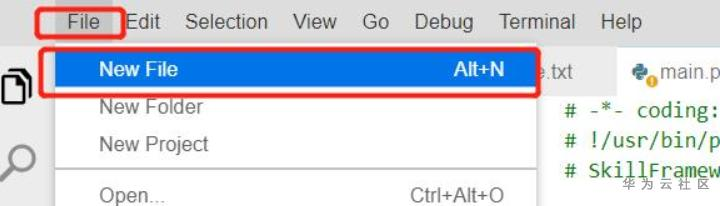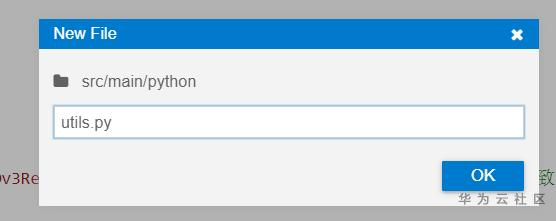```# -*- coding: utf-8 -*-
# !/usr/bin/python3

import cv2
import math
import numpy as np

# 检测模型输入尺寸
net_h = 352
net_w = 640

# 检测模型的类别
class_names = ["person"]
class_num   = len(class_names)

# 检测模型的anchors，用于解码出检测框
stride_list = [8, 16, 32]
anchors_1   = np.array([[10,13],   [16,30],   [33,23]])   / stride_list
anchors_2   = np.array([[30,61], [62,45],   [59,119]])   / stride_list
anchors_3   = np.array([[116,90], [156,198], [163,326]]) / stride_list
anchor_list = [anchors_1, anchors_2, anchors_3]

# 检测框的输出阈值、NMS筛选阈值和人形/人脸区域匹配阈值
conf_threshold   = 0.3
iou_threshold    = 0.4
cover_threshold  = 0.8

# 图片预处理：缩放到模型输入尺寸
def preprocess(img_data):
h, w, c   = img_data.shape
new_image = cv2.resize(img_data, (net_w, net_h))
return new_image, w, h

def overlap(x1, x2, x3, x4):
left  = max(x1, x3)
right = min(x2, x4)
return right - left

# 计算两个矩形框的IOU
def cal_iou(box1, box2):
w = overlap(box1, box1, box2, box2)
h = overlap(box1, box1, box2, box2)
if w <= 0 or h <= 0:
return 0
inter_area = w * h
union_area = (box1 - box1) * (box1 - box1) + (box2 - box2) * (box2 - box2) - inter_area
return inter_area * 1.0 / union_area

# 计算两个矩形框的IOU与box2区域的比值
def cover_ratio(box1, box2):
w = overlap(box1, box1, box2, box2)
h = overlap(box1, box1, box2, box2)
if w <= 0 or h <= 0:
return 0
inter_area = w * h
small_area = (box2 - box2) * (box2 - box2)
return inter_area * 1.0 / small_area

# 使用NMS筛选检测框
def apply_nms(all_boxes, thres):
res = []

for cls in range(class_num):
cls_bboxes   = all_boxes[cls]
sorted_boxes = sorted(cls_bboxes, key=lambda d: d)[::-1]

p = dict()
for i in range(len(sorted_boxes)):
if i in p:
continue

truth = sorted_boxes[i]
for j in range(i+1, len(sorted_boxes)):
if j in p:
continue
box = sorted_boxes[j]
iou = cal_iou(box, truth)
if iou >= thres:
p[j] = 1

for i in range(len(sorted_boxes)):
if i not in p:
res.append(sorted_boxes[i])
return res

# 从模型输出的特征矩阵中解码出检测框的位置、类别、置信度等信息
def decode_bbox(conv_output, anchors, img_w, img_h):

def _sigmoid(x):
s = 1 / (1 + np.exp(-x))
return s

_, h, w = conv_output.shape
pred    = conv_output.transpose((1,2,0)).reshape((h * w, 3, 5+class_num))

pred[..., 4:] = _sigmoid(pred[..., 4:])
pred[..., 0]  = (_sigmoid(pred[..., 0]) + np.tile(range(w), (3, h)).transpose((1,0))) / w
pred[..., 1]  = (_sigmoid(pred[..., 1]) + np.tile(np.repeat(range(h), w), (3, 1)).transpose((1,0))) / h
pred[..., 2]  = np.exp(pred[..., 2]) * anchors[:, 0:1].transpose((1,0)) / w
pred[..., 3]  = np.exp(pred[..., 3]) * anchors[:, 1:2].transpose((1,0)) / h

bbox          = np.zeros((h * w, 3, 4))
bbox[..., 0]  = np.maximum((pred[..., 0] - pred[..., 2] / 2.0) * img_w, 0)     # x_min
bbox[..., 1]  = np.maximum((pred[..., 1] - pred[..., 3] / 2.0) * img_h, 0)     # y_min
bbox[..., 2]  = np.minimum((pred[..., 0] + pred[..., 2] / 2.0) * img_w, img_w) # x_max
bbox[..., 3]  = np.minimum((pred[..., 1] + pred[..., 3] / 2.0) * img_h, img_h) # y_max

pred[..., :4] = bbox
pred          = pred.reshape((-1, 5+class_num))
pred[:, 4]    = pred[:, 4] * pred[:, 5:].max(1)    # 类别
pred          = pred[pred[:, 4] >= conf_threshold]
pred[:, 5]    = np.argmax(pred[:, 5:], axis=-1)    # 置信度

all_boxes = [[] for ix in range(class_num)]
for ix in range(pred.shape):
box = [int(pred[ix, iy]) for iy in range(4)]
box.append(int(pred[ix, 5]))
box.append(pred[ix, 4])
all_boxes[box-1].append(box)

return all_boxes

# 从模型输出中得到检测框
def get_result(model_outputs, img_w, img_h):

num_channel = 3 * (class_num + 5)
all_boxes   = [[] for ix in range(class_num)]
for ix in range(3):
pred      = model_outputs[2-ix].reshape((num_channel, net_h // stride_list[ix], net_w // stride_list[ix]))
anchors   = anchor_list[ix]
boxes     = decode_bbox(pred, anchors, img_w, img_h)
all_boxes = [all_boxes[iy] + boxes[iy] for iy in range(class_num)]

res = apply_nms(all_boxes, iou_threshold)
return res

# 在图中画出检测框，输出类别信息，注意这里对person类别绘制矩形框
def draw_boxes(img_data, bboxes):
thickness      = 2
font_scale     = 1
text_font      = cv2.FONT_HERSHEY_DUPLEX
for bbox in bboxes:
label = int(bbox)
x_min = int(bbox)
y_min = int(bbox)
x_max = int(bbox)
y_max = int(bbox)
score = bbox

# 1: person 蓝色
if label == 0:
# print(x_min, y_min, x_max, y_max)
cv2.rectangle(img_data, (x_min, y_min), (x_max, y_max), (0, 0, 255), thickness)
# cv2.putText(img_data, 'person', (x_min, y_min - 20), text_font, font_scale, (255, 255, 0), thickness)
# cv2.putText(img_data, score, (50, 50), text_font, font_scale, (255, 255, 0), thickness)

# 2:
'''
if label == 1:
# print(x_min, y_min, x_max, y_max)
cv2.rectangle(img_data, (x_min, y_min), (x_max, y_max), (255, 0, 0), thickness)
# cv2.putText(img_data, 'person', (x_min, y_min - 20), text_font, font_scale, (255, 255, 0), thickness)
# cv2.putText(img_data, score, (50, 50), text_font, font_scale, (255, 255, 0), thickness)

else:
# print("[INFO] Hi, find others.")
pass
'''

return img_data```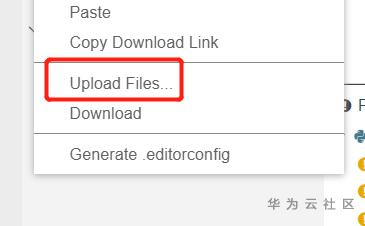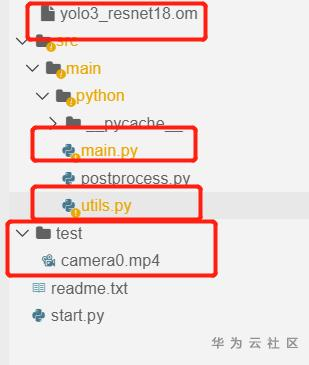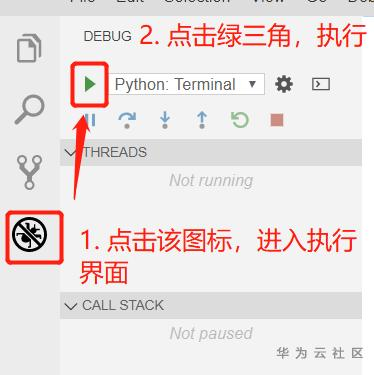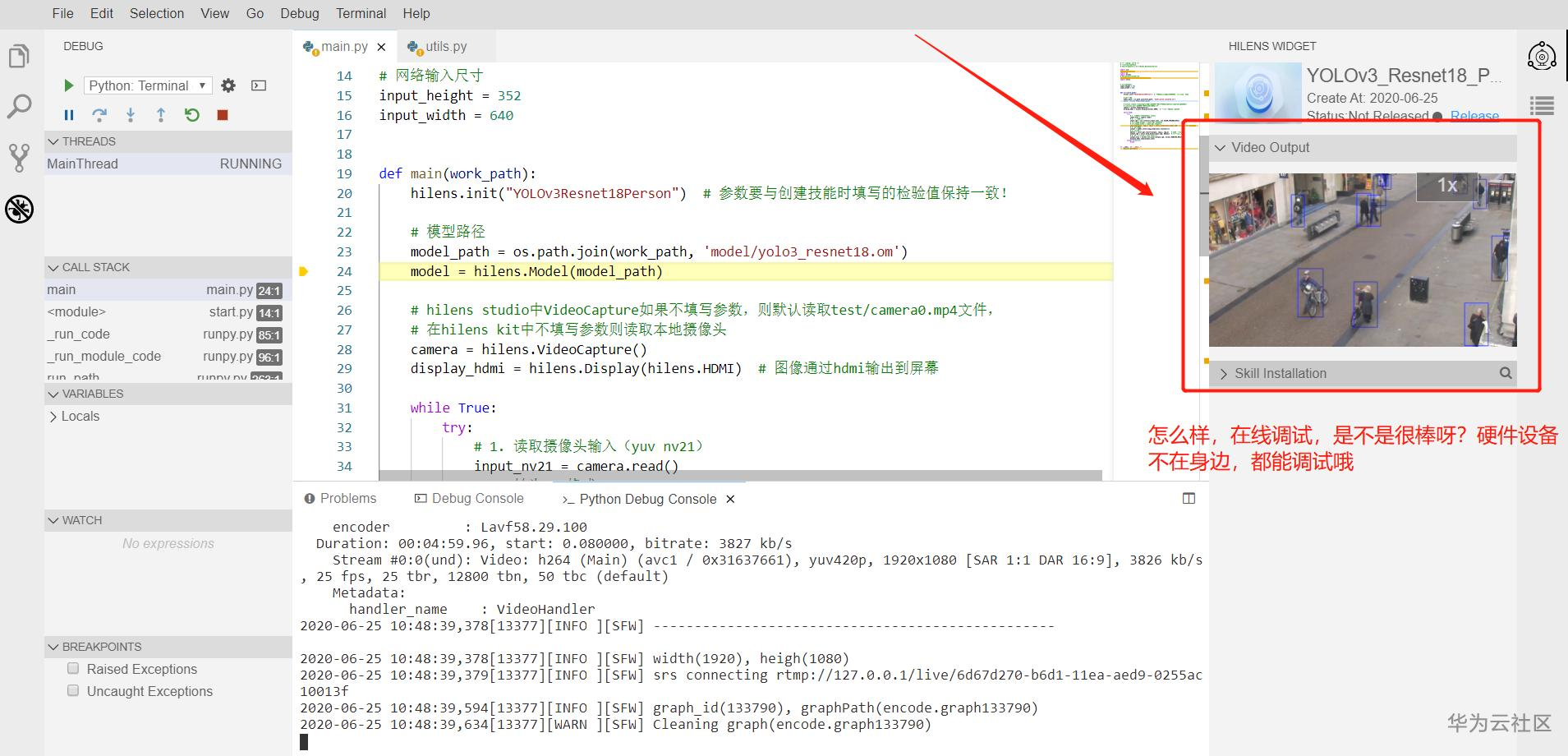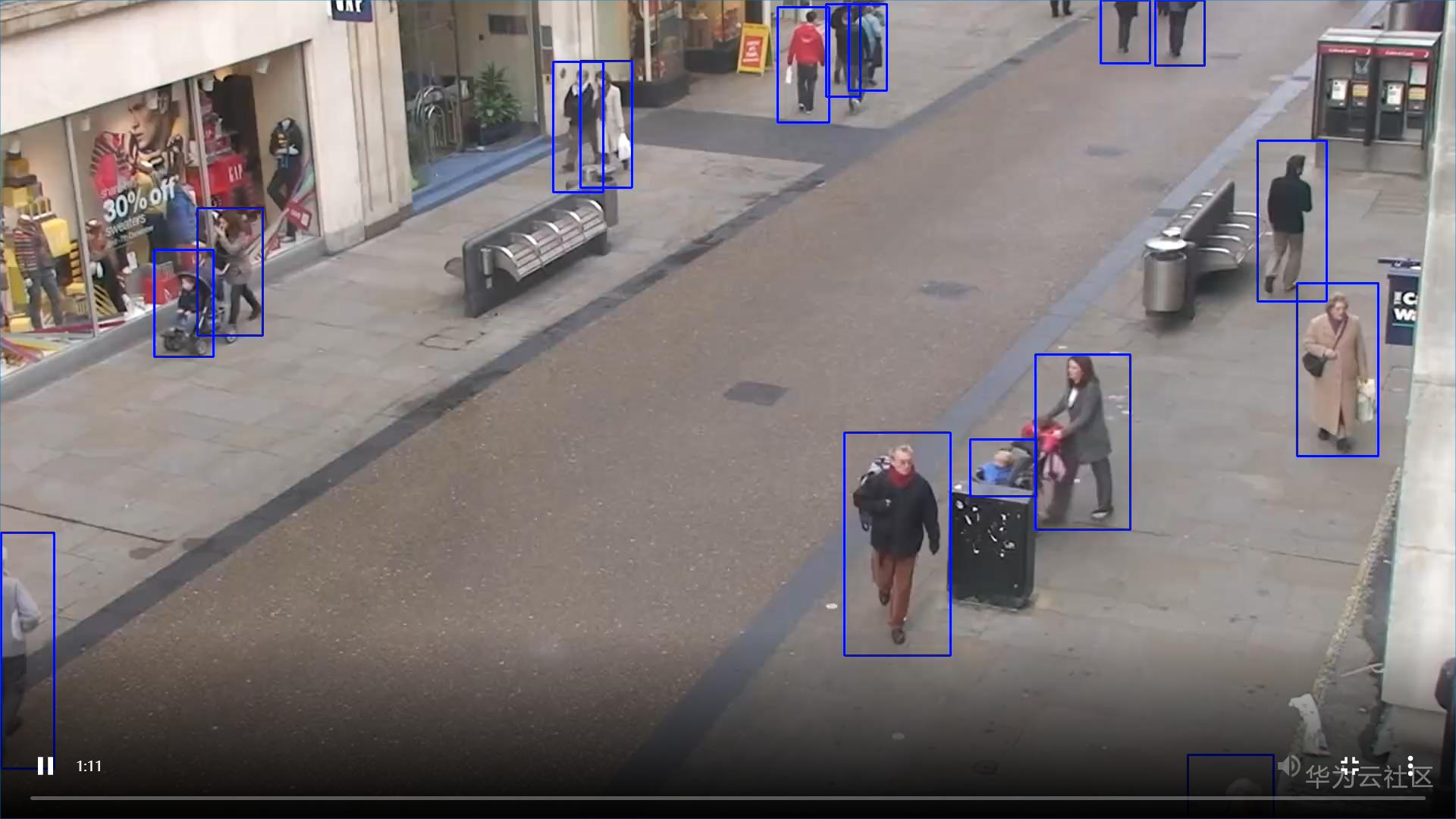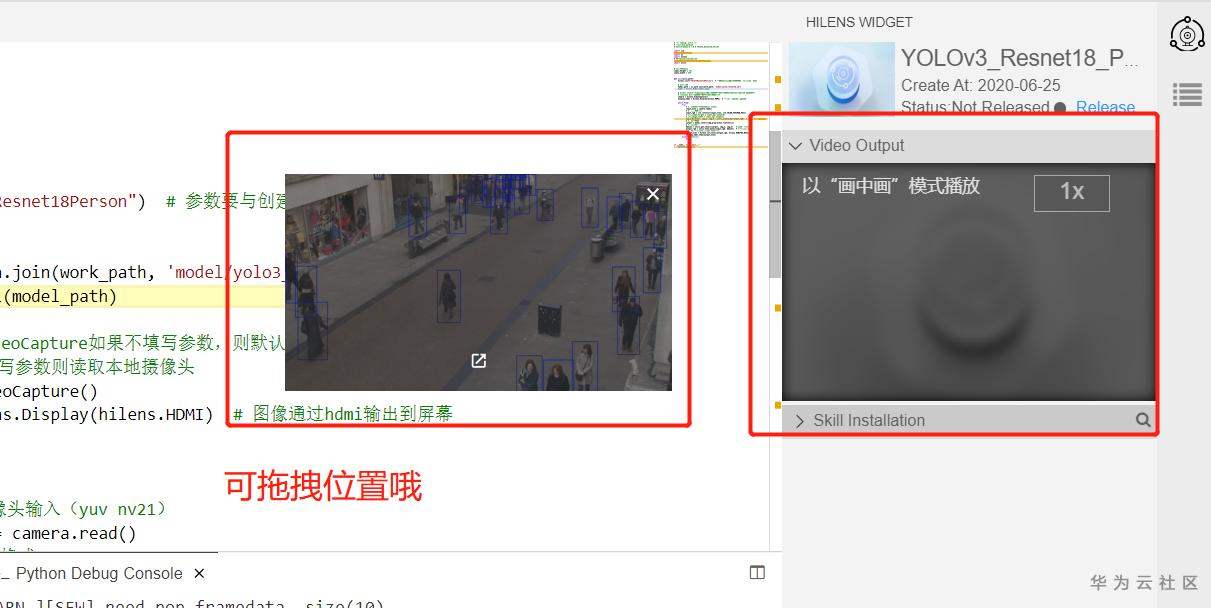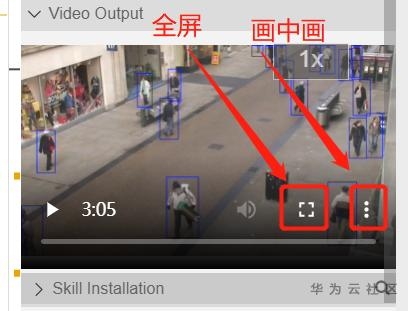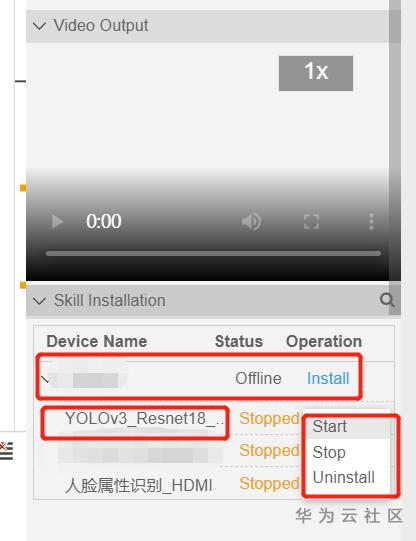【版权声明】本文为华为云社区用户原创内容，转载时必须标注文章的来源（华为云社区），文章链接，文章作者等基本信息，否则作者和本社区有权追究责任。如果您发现本社区中有涉嫌抄袭的内容，欢迎发送邮件至：huaweicloud.bbs@huawei.com进行举报，并提供相关证据，一经查实，本社区将立刻删除涉嫌侵权内容。

### 评论 (10)

0/1000

0/1000

0/1000

1. 重新训练模型；
2. 修改utils.py里的类别，及相应后处理
0/1000

###### 温馨提示